Function Repository Resource:

# NextKSubset

Get the lexicographic successor of a k-subset of a list

Contributed by: Wolfram Staff (original content by Sriram V. Pemmaraju and Steven S. Skiena)
 ResourceFunction["NextKSubset"][l,s] gives the k-subset of list l that follows the k-subset s in lexicographic order.

## Examples

### Basic Examples (1)

The lexicographic successor of a k-subset of an integer range:

 In:=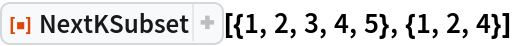Out=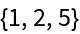### Properties and Relations (2)

NextKSubset[l,s] gives the successor to s in the list of k-subsets (where k is the length of s):

 In:=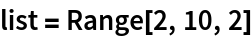Out=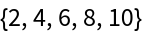In:=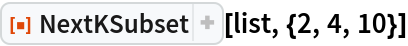Out=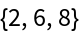In:=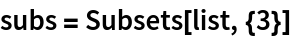Out=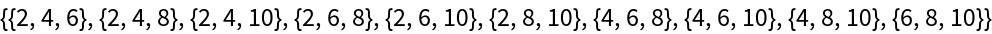The full list of subsets can also be obtained by iteratively applying NextKSubset, beginning at the initial such subset:

 In:=Out=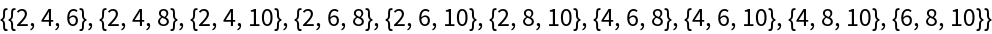In:=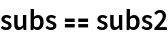Out=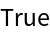## Version History

• 1.0.0 – 04 March 2020